# Introduction to Hashing – Data Structure and Algorithm Tutorials

• Difficulty Level : Medium
• Last Updated : 14 Sep, 2022

Hashing refers to the process of generating a fixed-size output from an input of variable size using the mathematical formulas known as hash functions. This technique determines an index or location for the storage of an item in a data structure.

## Need for Hash data structure

Every day, the data on the internet is increasing multifold and it is always a struggle to store this data efficiently. In day-to-day programming, this amount of data might not be that big, but still, it needs to be stored, accessed, and processed easily and efficiently. A very common data structure that is used for such a purpose is the Array data structure.

Now the question arises if Array was already there, what was the need for a new data structure! The answer to this is in the word “efficiency“. Though storing in Array takes O(1) time, searching in it takes at least O(log n) time. This time appears to be small, but for a large data set, it can cause a lot of problems and this, in turn, makes the Array data structure inefficient.

So now we are looking for a data structure that can store the data and search in it in constant time, i.e. in O(1) time. This is how Hashing data structure came into play. With the introduction of the Hash data structure, it is now possible to easily store data in constant time and retrieve them in constant time as well.

## Components of Hashing

There are majorly three components of hashing:

1. Key: A Key can be anything string or integer which is fed as input in the hash function the technique that determines an index or location for storage of an item in a data structure.
2. Hash Function: The hash function receives the input key and returns the index of an element in an array called a hash table. The index is known as the hash index.
3. Hash Table: Hash table is a data structure that maps keys to values using a special function called a hash function. Hash stores the data in an associative manner in an array where each data value has its own unique index.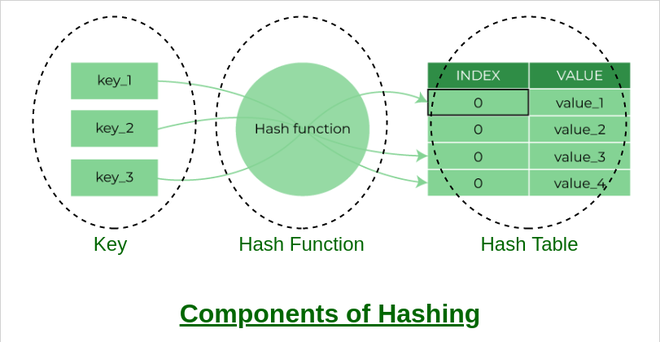Components of Hashing

## How does Hashing work?

Suppose we have a set of strings {“ab”, “cd”, “efg”} and we would like to store it in a table.

Our main objective here is to search or update the values stored in the table quickly in O(1) time and we are not concerned about the ordering of strings in the table. So the given set of strings can act as a key and the string itself will act as the value of the string but how to store the value corresponding to the key?

• Step 1: We know that hash functions (which is some mathematical formula) are used to calculate the hash value which acts as the index of the data structure where the value will be stored.
• Step 2: So, let’s assign
• “a” = 1,
• “b”=2, .. etc, to all alphabetical characters.
• Step 3: Therefore, the numerical value by summation of all characters of the string:
• “ab” = 1 + 2 = 3,
• “cd” = 3 + 4 = 7 ,
• “efg” = 5 + 6 + 7 = 18
• Step 4: Now, assume that we have a table of size 7 to store these strings. The hash function that is used here is the sum of the characters in key mod Table size. We can compute the location of the string in the array by taking the sum(string) mod 7.
• Step 5: So we will then store
• “ab” in 3 mod 7 = 3,
• “cd” in 7 mod 7 = 0, and
• “efg” in 18 mod 7 = 4.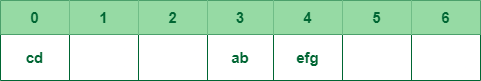Mapping key with indices of array

The above technique enables us to calculate the location of a given string by using a simple hash function and rapidly find the value that is stored in that location. Therefore the idea of hashing seems like a great way to store (key, value) pairs of the data in a table.

## What is a Hash function?

The hash function creates a mapping between key and value, this is done through the use of mathematical formulas known as hash functions. The result of the hash function is referred to as a hash value or hash. The hash value is a representation of the original string of characters but usually smaller than the original.

For example: Consider an array as a Map where the key is the index and the value is the value at that index. So for an array A if we have index i which will be treated as the key then we can find the value by simply looking at the value at A[i].
simply looking up A[i].

### Types of Hash functions:

There are many hash functions that use numeric or alphanumeric keys. This article focuses on discussing different hash functions:

### Properties of a Good hash function

A hash function that maps every item into its own unique slot is known as a perfect hash function. We can construct a perfect hash function if we know the items and the collection will never change but the problem is that there is no systematic way to construct a perfect hash function given an arbitrary collection of items. Fortunately, we will still gain performance efficiency even if the hash function isn’t perfect. We can achieve a perfect hash function by increasing the size of the hash table so that every possible value can be accommodated. As a result, each item will have a unique slot. Although this approach is feasible for a small number of items, it is not practical when the number of possibilities is large.

So, We can construct our hash function to do the same but the things that we must be careful about while constructing our own hash function.

A good hash function should have the following properties:

1. Efficiently computable.
2.  Should uniformly distribute the keys (Each table position is equally likely for each.
3. Should minimize collisions.
4. Should have a low load factor(number of items in the table divided by the size of the table).

### Complexity of calculating hash value using the hash function

• Time complexity: O(n)
• Space complexity: O(1)

## Problem with Hashing

If we consider the above example, the hash function we used is the sum of the letters, but if we examined the hash function closely then the problem can be easily visualized that for different strings same hash value is begin generated by the hash function.

For example: {“ab”, “ba”} both have the same hash value, and string {“cd”,”be”} also generate the same hash value, etc. This is known as collision and it creates problem in searching, insertion, deletion, and updating of value.

## What is collision?

The hashing process generates a small number for a big key, so there is a possibility that two keys could produce the same value. The situation where the newly inserted key maps to an already occupied, and it must be handled using some collision handling technology.

## How to handle Collisions?

There are mainly two methods to handle collision:

1. Separate Chaining: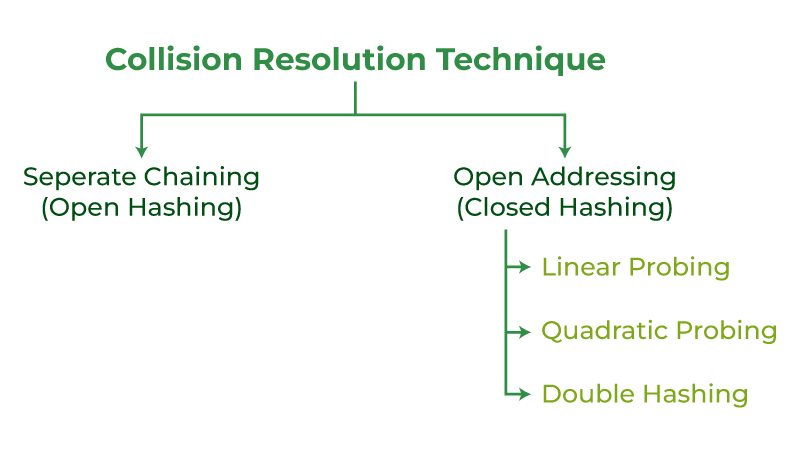Collision resolution technique

### 1) Separate Chaining

The idea is to make each cell of the hash table point to a linked list of records that have the same hash function value. Chaining is simple but requires additional memory outside the table.

Example: We have given a hash function and we have to insert some elements in the hash table using a separate chaining method for collision resolution technique.

```Hash function = key % 5,
Elements = 12, 15, 22, 25 and 37.```

Let’s see step by step approach to how to solve the above problem:

• Step 1: First draw the empty hash table which will have a possible range of hash values from 0 to 4 according to the hash function provided.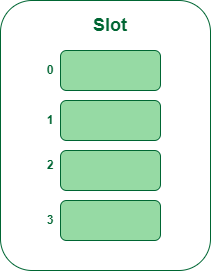Hash table

• Step 2: Now insert all the keys in the hash table one by one. The first key to be inserted is 12 which is mapped to bucket number 2 which is calculated by using the hash function 12%5=2.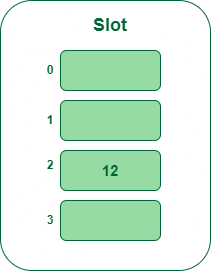Insert key 12 in the hash table

• Step 3: The next key is 15. It will map to slot number 0 because 15%5=0. So insert it into bucket number 5.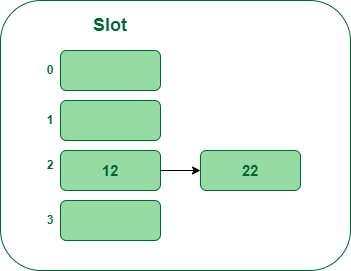Insert key 15 in the hash table

• Step 4: Now the next key is 22.  It will map to bucket number 2 because 22%5=2.  But bucket 2 is already occupied by key 12. So separate chaining method will handle this collision by creating a linked list to bucket 2.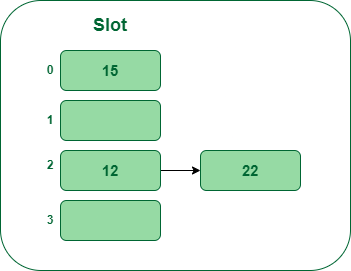Insert key 22 in the hash table

• Step 5: Now the next key is 25. Its bucket number will be 25%5=0. But bucket 0 is already occupied by key 25. So separate chaining method will again handle the collision by creating a linked list to bucket 0.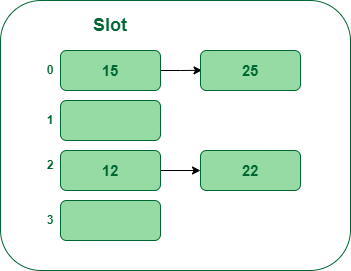Insert key 25 in the hash table

Hence In this way, the separate chaining method is used as the collision resolution technique.

### 2) Open Addressing

In open addressing, all elements are stored in the hash table itself. Each table entry contains either a record or NIL. When searching for an element, we examine the table slots one by one until the desired element is found or it is clear that the element is not in the table.

### 2.a) Linear Probing

In linear probing, the hash table is searched sequentially that starts from the original location of the hash. If in case the location that we get is already occupied, then we check for the next location.

Algorithm:

1. Calculate the hash key. i.e. key = data % size
2. Check, if hashTable[key] is empty
• store the value directly by hashTable[key] = data
3. If the hash index already has some value then
1.  check for next index using key = (key+1) % size
4. Check, if the next index is available hashTable[key] then store the value. Otherwise try for next index.
5. Do the above process till we find the space.

Example: Let us consider a simple hash function as “key mod 5” and a sequence of keys that are to be inserted are 50, 70, 76, 85, 93.

• Step1: First draw the empty hash table which will have a possible range of hash values from 0 to 4 according to the hash function provided.Hash table

• Step 2: Now insert all the keys in the hash table one by one. The first key is 50. It will map to slot number 0 because 50%5=0. So insert it into slot number 0.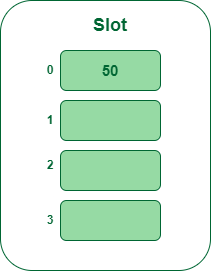Insert key 50 in the hash table

• Step 3: The next key is 70. It will map to slot number 0 because 70%5=0 but 50 is already at slot number 0 so, search for the next empty slot and insert it.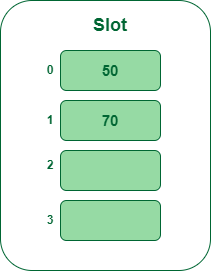Insert key 70 in the hash table

• Step 4: The next key is 76. It will map to slot number 1 because 76%5=1 but 70 is already at slot number 1 so, search for the next empty slot and insert it.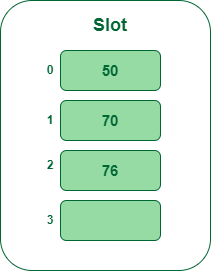Insert key 76 in the hash table

• Step 5: The next key is 93 It will map to slot number 3 because 93%5=3, So insert it into slot number 3.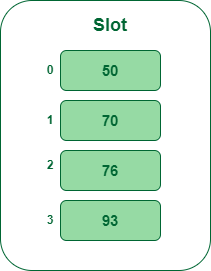Insert key 93 in the hash table

### 2.b) Quadratic Probing

Quadratic probing is an open addressing scheme in computer programming for resolving hash collisions in hash tables. Quadratic probing operates by taking the original hash index and adding successive values of an arbitrary quadratic polynomial until an open slot is found.

An example sequence using quadratic probing is:

H + 12, H + 22, H + 32, H + 42…………………. H + k2

This method is also known as the mid-square method because in this method we look for i2‘th probe (slot) in i’th iteration and the value of i = 0, 1, . . . n – 1. We always start from the original hash location. If only the location is occupied then we check the other slots.

Let hash(x) be the slot index computed using the hash function and n be the size of the hash table.

If the slot hash(x) % n is full, then we try (hash(x) + 12) % n.
If (hash(x) + 12) % n is also full, then we try (hash(x) + 22) % n.
If (hash(x) + 22) % n is also full, then we try (hash(x) + 32) % n.
This process will be repeated for all the values of i until an empty slot is found

Example: Let us consider table Size = 7, hash function as Hash(x) = x % 7 and collision resolution strategy to be f(i) = i2 . Insert = 25, 33, and 105

• Step 1: Create a table of size 7.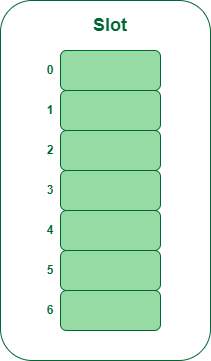Hash table

• Step 2 – Insert 22 and 30
• Hash(25) = 22 % 7 = 1, Since the cell at index 1 is empty, we can easily insert 22 at slot 1.
• Hash(30) = 30 % 7 = 2, Since the cell at index 2 is empty, we can easily insert 30 at slot 2.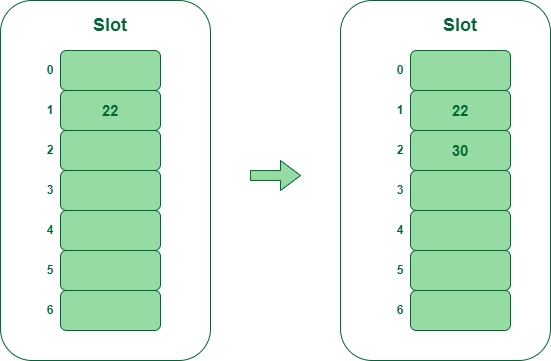Insert key 22 and 30 in the hash table

• Step 3: Inserting 50
• Hash(25) = 50 % 7 = 1
• In our hash table slot 1 is already occupied. So, we will search for slot 1+12, i.e. 1+1 = 2,
• Again slot 2 is found occupied, so we will search for cell 1+22, i.e.1+4 = 5,
• Now, cell 5 is not occupied so we will place 50 in slot 5.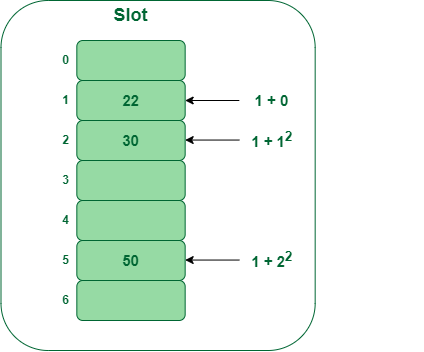Insert key 50 in the hash table

### 2.c) Double Hashing

Double hashing is a collision resolving technique in Open Addressed Hash tables. Double hashing make use of two hash function,

• The first hash function is h1(k) which takes the key and gives out a location on the hash table. But if the new location is not occupied or empty then we can easily place our key.
• But in case the location is occupied (collision) we will use secondary hash-function h2(k) in combination with the first hash-function h1(k) to find the new location on the hash table.

This combination of hash functions is of the form

`h(k, i) = h1(k) + i * h2(k)) % n `

where

• i is a non-negative integer that indicates a collision number,
• k = element/key which is being hashed
• n = hash table size.

Complexity of the Double hashing algorithm:

`Time complexity: O(n)`

Example: Insert the keys 27, 43, 98, 72 into the Hash Table of size 7. where first hash-function is h1​(k) = k mod 7 and second hash-function is h2(k) = 1 + (k mod 5)

• Step 1: Insert 27
• 27 % 7 = 6, location 6 is empty so insert 27 into 6 slot.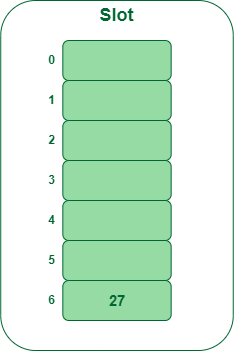Insert key 27 in the hash table

• Step 2: Insert 43
• 43 % 7 = 1, location 1 is empty so insert 43 into 1 slot.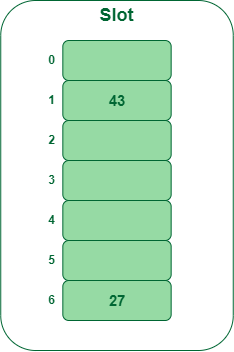Insert key 43 in the hash table

• Step 3: Insert 92
• 92 % 7 = 6, but location 6 is already being occupied and this is a collision
• So we need to resolve this collision using double hashing.
```hnew = [h1(92) + i * (h2(92)] % 7
= [6 + 1 * (1 + 92 % 5)] % 7
= 9 % 7
= 2

Now, as 2 is an empty slot,
so we can insert 92 into 2nd slot.```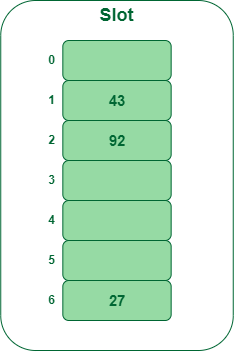Insert key 92 in the hash table

• Step 4: Insert 72
• 72 % 7 = 2, but location 2 is already being occupied and this is a collision.
• So we need to resolve this collision using double hashing.
```hnew = [h1(72) + i * (h2(72)] % 7
= [2 + 1 * (1 + 72 % 5)] % 7
= 5 % 7
= 5,

Now, as 5 is an empty slot,
so we can insert 72 into 5th slot.```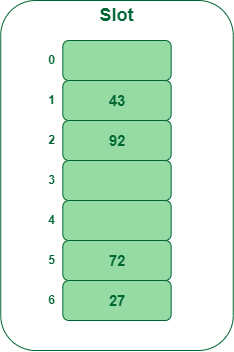Insert key 72 in the hash table

## What is meant by Load Factor in Hashing?

The load factor of the hash table can be defined as the number of items the hash table contains divided by the size of the hash table. Load factor is the decisive parameter that is used when we want to rehash the previous hash function or want to add more elements to the existing hash table.

It helps us in determining the efficiency of the hash function i.e. it tells whether the hash function which we are using is distributing the keys uniformly or not in the hash table.

`Load Factor = Total elements in hash table/ Size of hash table `

## What is Rehashing?

As the name suggests, rehashing means hashing again. Basically, when the load factor increases to more than its predefined value (the default value of the load factor is 0.75), the complexity increases. So to overcome this, the size of the array is increased (doubled) and all the values are hashed again and stored in the new double-sized array to maintain a low load factor and low complexity.

## Applications of Hash Data structure

• Hash is used in databases for indexing.
• Hash is used in disk-based data structures.
• In some programming languages like Python, JavaScript hash is used to implement objects.

## Real-Time Applications of Hash Data structure

• Hash is used for cache mapping for fast access to the data.
• Hash can be used for password verification.
• Hash is used in cryptography as a message digest.

## Advantages of Hash Data structure

• Hash provides better synchronization than other data structures.
• Hash tables are more efficient than search trees or other data structures
• Hash provides constant time for searching, insertion, and deletion operations on average.

## Disadvantages of Hash Data structure

• Hash is inefficient when there are many collisions.
• Hash collisions are practically not avoided for a large set of possible keys.
• Hash does not allow null values.

## Conclusion

From the above discussion, we conclude that the goal of hashing is to resolve the challenge of finding an item quickly in a collection. For example, if we have a list of millions of English words and we wish to find a particular term then we would use hashing to locate and find it more efficiently. It would be inefficient to check each item on the millions of lists until we find a match. Hashing reduces search time by restricting the search to a smaller set of words at the beginning.

My Personal Notes arrow_drop_up
Recommended Articles
Page :